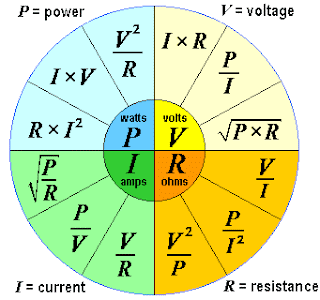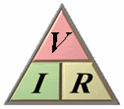## PAGINAS

### Calculadora Lei de Ohm

Formula wheel electrical engineering electricity ohm's law pie chart calculation circle power wheel electric power formula fundamentals general ohm's law emf ohms audio physics electricity electronics formula wheel formulas amps watts volts ohms cosine equation audio engineering pie chart charge physics formula for power calc voltage bridging - sengpielaudio Sengpiel Berlin
Formulas for calculating any combination of electrical units

 Principles of Electrical Engineering Physics Formulary and Electricity Equations Formula Wheel ▼ Important Formulas Electrical engineering laws Electronic engineering laws Electrical formulas as circle diagram (pie chart)V comes from "voltage" and E from "electromotive force emf". E means also energy, so let's choose V. Energy = voltage × charge. E = V × Q. Some like better to stick to E instead to V, so do it.

 Voltage V = I × R = P / I = √(P × R) in volts V          Current I = V / R = P / V = √(P / R) in amperes A Resistance R = V / I = P / I2 = V2/ P in ohms Ω      Power P = V × I = R × I2= V2/ R in watts W

Electric voltage V = I × R      (Ohm's law formula)
Electric voltage = amperage × resistance

Please enter two values, the third value will be calculated.

 Electric voltage V voltsAmperage I amps Resistance R ohms V = I × R              I = V ⁄ R              R = V ⁄ I
Electric power P = I × V      (Watt's power law formula)
Electric power = amperage × voltage

Please enter two values, the third value will be calculated.

 Electric Power P wattsAmperage I amps Voltage V volts P = I × V              I = P ⁄ V              V = P ⁄ I

If the unit of power P = I × V and of voltage V = I · R is needed
look for "The Big Power Formulas":
Calculations: Power (watt), voltage, current, and resistance